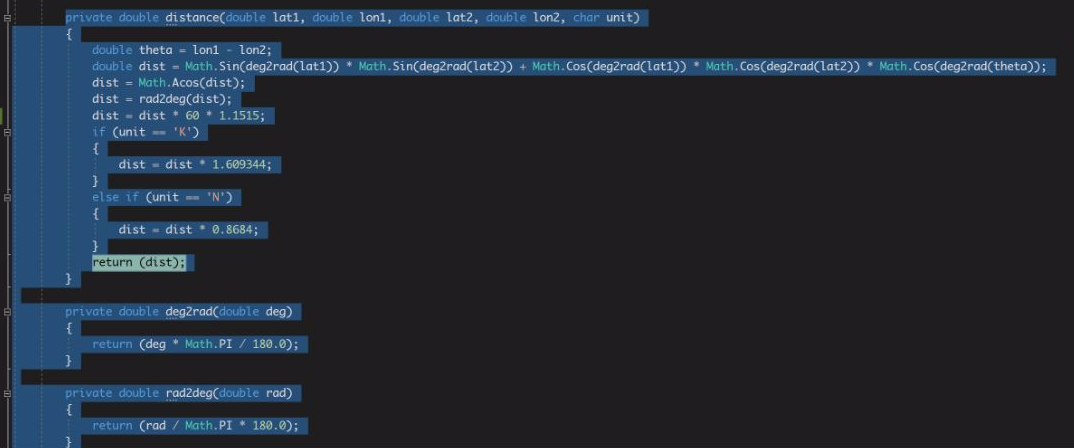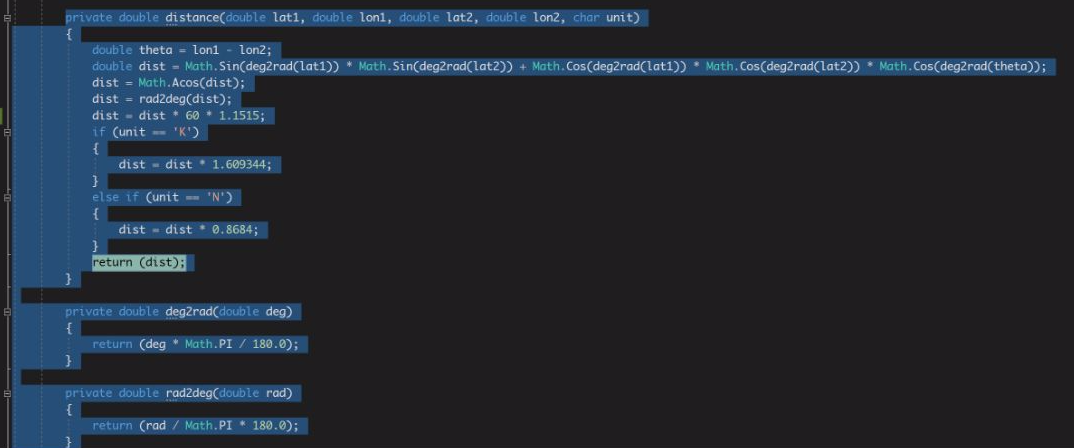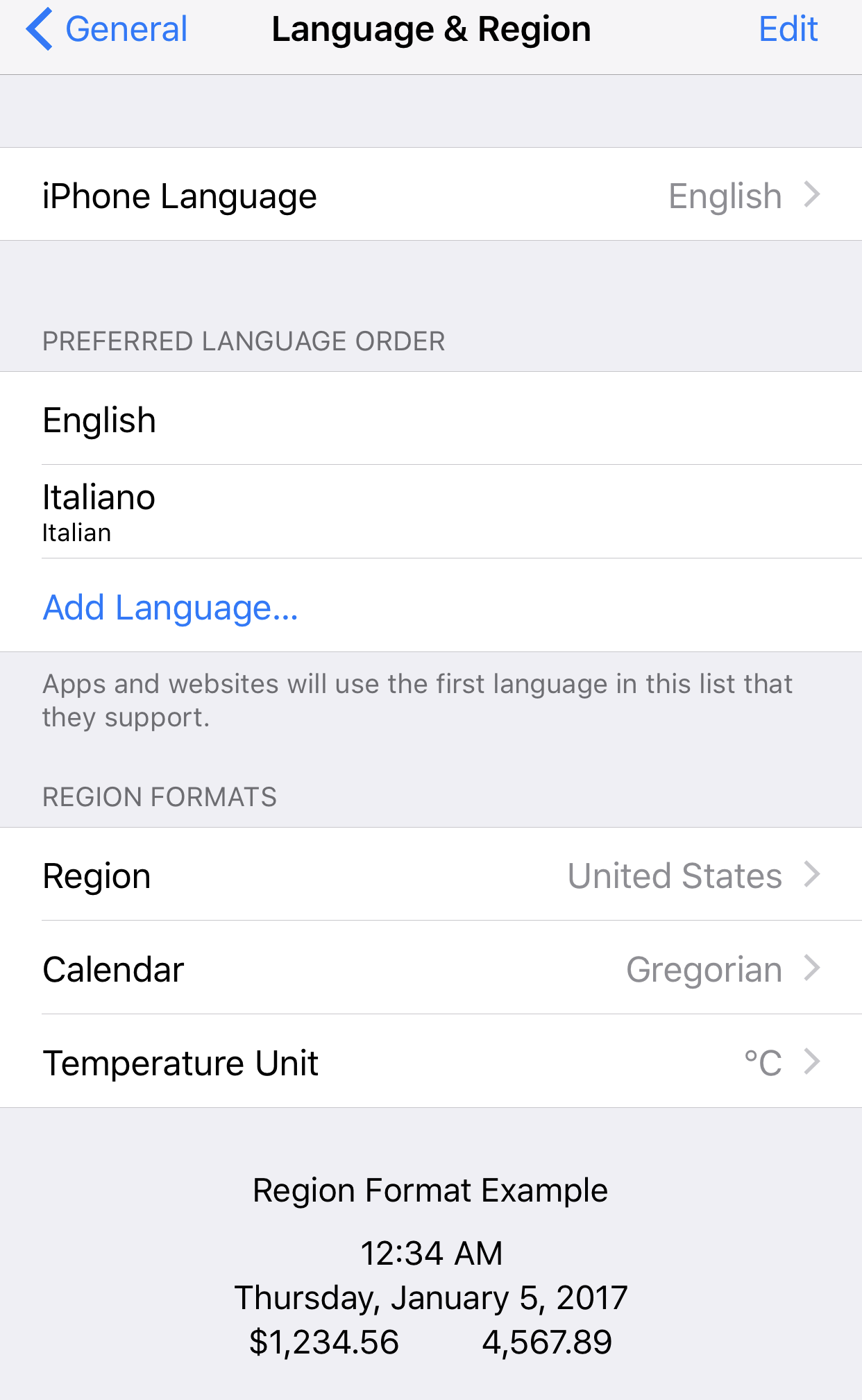# Math operations: different results from iOS emulator to real device.Maybe culture problems?

Hi,
I create an app with some calculation on distance between geoloc points.
On my emulator all works ( Region and Language are: Language English and Region United States ), but on my device ( region and language are italian ) the results are wrong.
I think a problem about of culture because I see in the app the number with the dot as comma that it the number are in US or EN culture.
How to manipulate the numbers independently by culture?

Tagged:

## Posts

• @biapar - All of the usual conversion methods have locale-specific and locale-independent options.

Converting a double to a string in a locale independent way is done by:

```value.ToString(CultureInfo.InvariantCulture)
```
• I find the problem, but not the solution.
This code return the correct results, if I setup my phone with English language and United States region. See images.• @biapar - Show the code where you take the double value and convert it to a string for display (which could be in a value converter or elsewhere).

Also. what result do you want? Do you want it to always use a "." for the decimal separator, or do you want it to use the appropriate character for the current locale?

• You can force a Culture on the app which would fix the problem

This would go in the platform specific code.

I wish to return the correct result by current locale. That is 1.2345,45 for ITA.

• @biapar - Show the code where you take the double value and convert it to a string for display (which could be in a value converter or elsewhere).

• Distance calculation:

private double distance(double lat1, double lon1, double lat2, double lon2, char unit)
{
double theta = lon1 - lon2;
dist = Math.Acos(dist);
dist = dist * 60 * 1.1515;
if (unit == 'K')
{
dist = dist * 1.609344;
}
else if (unit == 'N')
{
dist = dist * 0.8684;
}
return (dist);
}

```    private double deg2rad(double deg)
{
return (deg * Math.PI / 180.0);
}

{
return (rad / Math.PI * 180.0);
}
```

Variable Assignment ( elemento.Distanza is a Double ):

elemento.Distanza = Math.Round(distance(lastmypoint.Latitude, lastmypoint.Longitude, pointB.Latitude, pointB.Longitude, 'K'),2);

• @biapar - Show the code where you take the double value and convert it to a string for display (which could be in a value converter or elsewhere).

The calculation code only operates on doubles. It's the code that takes the doubles and goes on to display them that is relevant here.

• @JohnHardman said:
@biapar - Show the code where you take the double value and convert it to a string for display (which could be in a value converter or elsewhere).

The calculation code only operates on doubles. It's the code that takes the doubles and goes on to display them that is relevant here.

I assign the value with this code:
elemento.Distanza = Math.Round(distance(lastmypoint.Latitude, lastmypoint.Longitude, pointB.Latitude, pointB.Longitude, 'K'),2);

and show the value with Label

• I solved the problem.
The problem was caused by dot into the item value and in Italian culture was used like thousand separator.

```Position pointB = new Position(double.Parse(item.Lat, CultureInfo.InvariantCulture), double.Parse(item.Long, CultureInfo.InvariantCulture));
```